# MCQs on Control Systems

##### Page 4 of 23. Go to page 1 2 3 4 5 6 7 8 9 10 11 12 13 14 15 16 17 18 19 20 21 22 23
01․ The transfer function for tachometer E(S)/θ(S) is
K
KS
K/S
KS2

Tachometer is a speed transducer. They are used as feedback elements in control system applications. DC tachometer is a small DC generator whose input is mechanical speed and output is electrical voltage, voltage is proportional to speed. Voltage E ∝ Speed (ω = dθ/dt) E = K dθ/dt or E = Kω By taking Laplace transforms, E(S) = KS θ(S) or E(S) = Kω(S) Transfer function E(S)/θ(S) = KS E(S)/ω(S) = K

02․ Transient state analysis deals with -------------
magnitude of error
nature of response
both 1 and 2
none of the above

Transient state analysis: It deals with the nature of response of the system when subjected to an input. Steady state analysis: It deals with the estimation of magnitude of steady state error between output and input.

03․ Open loop transfer function,all pass filter.
band stop filter.
band reject filter.
none of the above.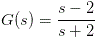This denotes a zero at s = 2 and a pole at s = - 2. So there exists a pole and a zero one at left half of s plane and other at right half of s plane. They denote symmetrical mirror images, so the given open loop transfer function denotes an all pass filter.

04․ When compared to a 1st order LPF, a 2nd order LPF has
lower voltage gain.
higher voltage gain.
higher cut off frequency.
faster drop in filter response.

2nd order low pass filter have higher cut off frequency than 1st order low pass filter.

05․ The step response of the system described by the differential equation will be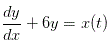(1/6)*(1-e-6t).
e-3t u(t).
e6t.
non of above.

Applying Laplace Transform,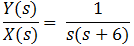Applying Inverse Laplace Transform, Y(t) = (1/6) × (1-e-6t)

06․ The impulse response of the system described by the differential equation will be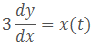(1/3) × (1-e-3t).
e-3t u(t).
1/3.
e6t.

Applying Laplace Transform,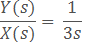Applying Inverse Laplace Transform, Y(t) = 1/3.

07․ The impulse response of the system described by the differential equation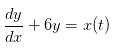will be
e6t.
e-3tu(t).
e-6t.
none of above.

Take Laplace transform above differential equation.

08․ The type of a control system is obtained from -------------- transfer function?
open loop
closed loop
both open loop and closed loop
none of above.

The type of the system is obtained from open loop transfer function. The number of open loop poles occurring at origin determines the type of the control system.

09․ What is the type of closed loop system for the plant transfer function G(s) = k/s²(1+Ts) and with unity feedback?
1
3
2
0

Every transfer function representing a control system has certain type and order. The steady state analysis depends on the type of the control system. The type of the system is obtained from open loop transfer function. The number of open loop poles occurring at origin determines the type of the control system. Therefore, for given transfer function type is 2.

10․ Which of the following statement is/are true statements?
Type of the system is obtained from open loop transfer function
The steady state analysis depends on type of the system
Transient state analysis depends on order of the system
all of the above

Every transfer function representing a control system has certain type and order. The transient state analysis depends on the order of the control system. The order of system is obtained from closed loop transfer function. The highest power of the characteristic equation (1 + G(s)H(s) )determines the order of the control system. The steady state analysis depends on the type of the control system. The type of the system is obtained from open loop transfer function. The number of open loop poles occurring at origin determines the type of the control system.

<<<23456>>>